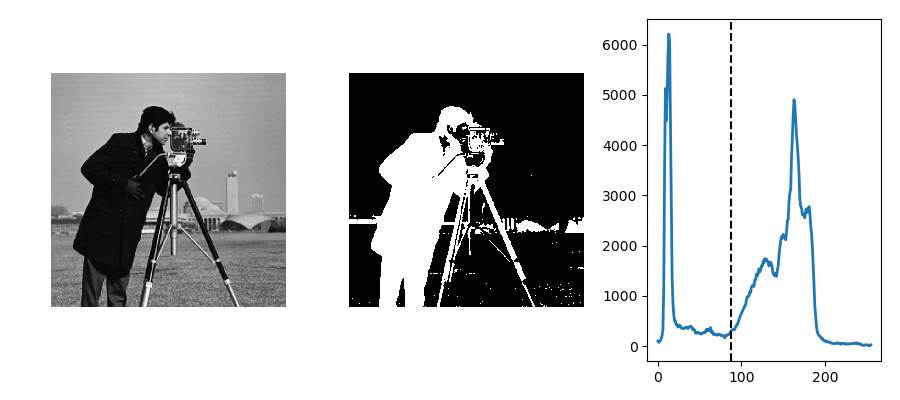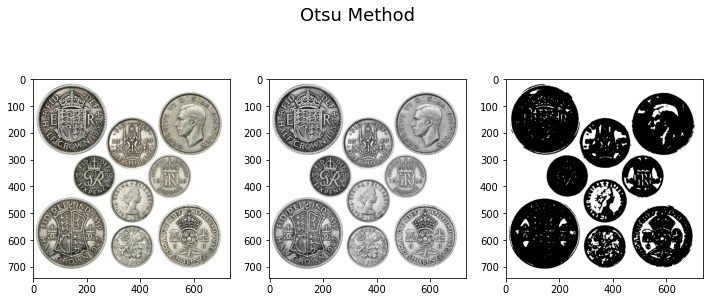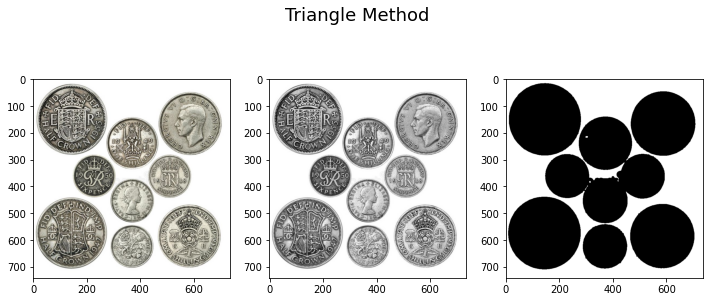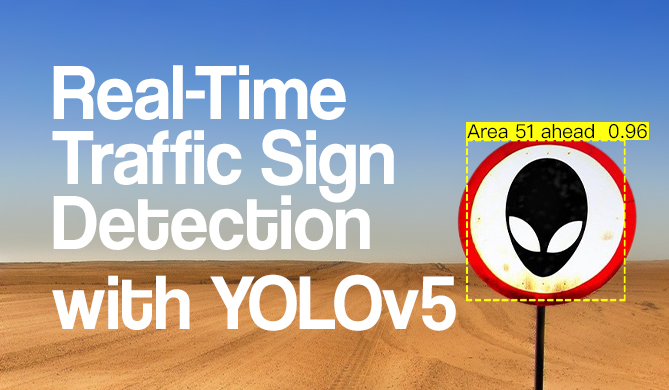OpenCV Thresholding in Python with cv2.threshold()

# OpenCV Thresholding in Python with cv2.threshold()## Introduction

Thresholding is a simple and efficient technique to perform basic segmentation in an image, and to binarize it (turn it into a binary image) where pixels are either 0 or 1 (or 255 if you're using integers to represent them).

Typically, you can use thresholding to perform simple background-foreground segmentation in an image, and it boils down to variants on a simple technique for each pixel:

if pixel_value > threshold:
pixel_value = MAX
else:
pixel_value = 0


This essential process is known as Binary Thresholding. Now - there are various ways you can tweak this general idea, including inverting the operations (switching the > sign with a < sign), setting the pixel_value to the threshold instead of a maximum value/0 (known as truncating), keeping the pixel_value itself if it's above the threshold or if it's below the threshold.

All of these have conveniently been implemented in OpenCV as:

• cv2.THRESH_BINARY
• cv2.THRESH_BINARY_INV
• cv2.THRESH_TRUNC
• cv2.THRESH_TOZERO
• cv2.THRESH_TOZERO_INV

... respectively. These are relatively "naive" methods in that they're fairly simple, don't account for context in images, have knowledge of what shapes are common, etc. For these properties - we'd have to employ much more computationally expensive and powerful techniques.Advice: If you'd like to learn more about multi-class semantic segmentation with Deep Learning - you can enroll our DeepLabV3+ Semantic Segmentation with Keras!

Now, even with the "naive" methods - some heuristics can be put into place, for finding good thresholds, and these include the Otsu method and the Triangle method:

• cv2.THRESH_OTSU
• cv2.THRESH_TRIANGLENote: OpenCV thresholding is a rudimentary technique, and is sensitive to lighting changes and gradients, color heterogeneity, etc. It's best applied on relatively clean pictures, after blurring them to reduce noise, without much color variance in the objects you want to segment.

Another way to overcome some of the issues with basic thresholding with a single threshold value is to use adaptive thresholding which applies a threshold value on each small region in an image, rather than globally.## Simple Thresholding with OpenCV

Thresholding in OpenCV's Python API is done via the cv2.threshold() method - which accepts an image (NumPy array, represented with integers), the threshold, maximum value and thresholding method (how the threshold and maximum_value are used):

img = cv2.imread('objects.jpg')
# Convert from BGR to RGB colorspace
img = cv2.cvtColor(img, cv2.COLOR_BGR2RGB)
# Blurring usually helps with ironing out small details that can
# make segmentation maps look full of 'specks'
blurred = cv2.GaussianBlur(img, (7, 7), 0)
# Run thresholding, returning the masked image and return code
ret, img_masked = cv2.threshold(blurred, 220, 255, cv2.THRESH_BINARY)


The return code is just the applied threshold:

print(f"Threshold: {ret}") # Threshold: 125


Here, since the threshold is 220 and we've used the THRESH_BINARY method - every pixel value above 220 will be increased to 255, while every pixel value below 220 will be lowered to 0, creating a black and white image, with a "mask", covering the foreground objects.

Why 220? Knowing what the image looks like allows you to make some approximate guesses about what threshold you can choose. In practice, you'll rarely want to set a manual threshold, and we'll cover automatic threshold selection in a moment.

Let's plot the result! OpenCV windows can be a bit finicky, so we'll plot the original image, blurred image and results using Matplotlib:

fig, ax = plt.subplots(1, 3, figsize=(12, 8))
ax.imshow(img)
ax.imshow(blurred)


### Thresholding Methods

As mentioned earlier, there are various ways you can use the threshold and maximum value in a function. We've taken a look at the binary threshold initially. Let's create a list of methods, and apply them one by one, plotting the results:

methods = [cv2.THRESH_BINARY, cv2.THRESH_BINARY_INV, cv2.THRESH_TRUNC, cv2.THRESH_TOZERO, cv2.THRESH_TOZERO_INV]
names = ['Binary Threshold', 'Inverse Binary Threshold', 'Truncated Threshold', 'To-Zero Threshold', 'Inverse To-Zero Threshold']

def thresh(img_path, method, index):
img = cv2.cvtColor(img, cv2.COLOR_BGR2RGB)
blurred = cv2.GaussianBlur(img, (7, 7), 0)
ret, img_masked = cv2.threshold(blurred, 220, 255, method)

fig, ax = plt.subplots(1, 3, figsize=(12, 4))
fig.suptitle(names[index], fontsize=18)
ax.imshow(img)
ax.imshow(blurred)
plt.tight_layout()

for index, method in enumerate(methods):
thresh('coins.jpeg', method, index)


THRESH_BINARY and THRESH_BINARY_INV are inverse of each other, and binarize an image between 0 and 255, assigning them to the background and foreground respectively, and vice versa.

THRESH_TRUNC "binarizes" the image between threshold and 255.

THRESH_TOZERO and THRESH_TOZERO_INV binarize between 0 and the current pixel value (src(x, y)). Let's take a look at the resulting images:

Free eBook: Git Essentials

Check out our hands-on, practical guide to learning Git, with best-practices, industry-accepted standards, and included cheat sheet. Stop Googling Git commands and actually learn it!

These methods are intuitive enough - but, how can we automate a good threshold value, and what does a "good threshold" value even mean? Most of the results so far had non-ideal masks, with marks and specks in them. This happens because of the difference in the reflective surfaces of the coins - they're not uniformly colored due to the difference in how ridges reflect light.

We can, to a degree, battle this by finding a better global threshold.

## Automatic/Optimized Thresholding with OpenCV

OpenCV employs two effective global threshold searching methods - Otsu's method, and the Triangle method.

Otsu's method assumes that it's working on bi-modal images. Bi-modal images are images whose color histograms only contain two peaks (i.e. has only two distinct pixel values). Considering that the peaks each belong to a class such as a "background" and "foreground" - the ideal threshold is right in the middle of them.Image credit: https://scipy-lectures.org/

You can make some images more bi-modal with gaussian blurs, but not all.

An alternative algorithm is the triangle algorithm, which calculates the distance between the maximum and minimum of the gray-level histogram and draws a line. The point at which that line is maximally far away from the rest of the histogram is chosen as the threshold:

There's no competition between them - they each work on different types of images, so it's best to try them out and see which returns the better result. Both of these assume a grayscale image, so we'll need to convert the input image to gray via cv2.cvtColor():

img = cv2.imread(img_path)
gray = cv2.cvtColor(img, cv2.COLOR_BGR2GRAY)
blurred = cv2.GaussianBlur(gray, (7, 7), 0)

ret, mask1 = cv2.threshold(blurred, 0, 255, cv2.THRESH_OTSU)
ret, mask2 = cv2.threshold(blurred, 0, 255, cv2.THRESH_TRIANGLE)
# ...


Let's run the image through with both methods and visualize the results:

methods = [cv2.THRESH_OTSU, cv2.THRESH_TRIANGLE]
names = ['Otsu Method', 'Triangle Method']

def thresh(img_path, method, index):
gray = cv2.cvtColor(img, cv2.COLOR_BGR2GRAY)
blurred = cv2.GaussianBlur(gray, (7, 7), 0)

ret, img_masked = cv2.threshold(blurred, 0, 255, method)
print(f"Threshold: {ret}")

fig, ax = plt.subplots(1, 3, figsize=(12, 5))
fig.suptitle(names[index], fontsize=18)
ax.imshow(cv2.cvtColor(img, cv2.COLOR_BGR2RGB))
ax.imshow(cv2.cvtColor(gray, cv2.COLOR_BGR2RGB))

for index, method in enumerate(methods):
thresh('coins.jpeg', method, index)Here, the triangle method outperforms Otsu's method, because the image isn't bi-modal:

import numpy as np

gray = cv2.cvtColor(img, cv2.COLOR_BGR2GRAY)
blurred = cv2.GaussianBlur(gray, (7, 7), 0)

histogram_gray, bin_edges_gray = np.histogram(gray, bins=256, range=(0, 255))
histogram_blurred, bin_edges_blurred = np.histogram(blurred, bins=256, range=(0, 255))

fig, ax = plt.subplots(1, 2, figsize=(12, 4))

ax.plot(bin_edges_gray[0:-1], histogram_gray)
ax.plot(bin_edges_blurred[0:-1], histogram_blurred)


However, it's clear how the triangle method was able to work with the image and produce a more satisfying result.

## Limitations of OpenCV Thresholding

Thresholding with OpenCV is simple, easy and efficient. Yet, it's fairly limited. As soon as you introduce colorful elements, non-uniform backgrounds and changing lighting conditions - global thresholding as a concept becomes too rigid.

Images are usually too complex for a single threshold to be enough, and this can partially be addressed through adaptive thresholding, where many local thresholds are applied instead of a single global one. While also limited, adaptive thresholding is much more flexible than global thresholding.

## Conclusion

In recent years, binary segmentation (like what we did here) and multi-label segmentation (where you can have an arbitrary number of classes encoded) has been successfully modeled with deep learning networks, which are much more powerful and flexible. In addition, they can encode global and local context into the images they're segmenting. The downside is - you need data to train them, as well as time and expertise.

For on-the-fly, simple thresholding, you can use OpenCV. For accurate, production-level segmentation, you'll want to use neural networks.

Last Updated: November 17th, 2023

Get tutorials, guides, and dev jobs in your inbox.

David LandupAuthor

Entrepreneur, Software and Machine Learning Engineer, with a deep fascination towards the application of Computation and Deep Learning in Life Sciences (Bioinformatics, Drug Discovery, Genomics), Neuroscience (Computational Neuroscience), robotics and BCIs.

Great passion for accessible education and promotion of reason, science, humanism, and progress.Project

### Real-Time Road Sign Detection with YOLOv5

# python# machine learning# computer vision# pytorch

If you drive - there's a chance you enjoy cruising down the road. A responsible driver pays attention to the road signs, and adjusts their...David Landup
DetailsProject

### Building Your First Convolutional Neural Network With Keras

# python# artificial intelligence# machine learning# tensorflow

Most resources start with pristine datasets, start at importing and finish at validation. There's much more to know. Why was a class predicted? Where was...David Landup
Details